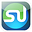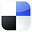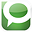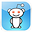# The t TestSimplified and Done in Excel

If you had recently launched a new marketing campaign, you would want to know as soon as possible whether the campaign was working. If you are able to take a large sample of before and after measurements (for example, in all of the sales territories), Excel has the perfect tool for you  a data analysis tool called the two-sample paired t-test for means. It is very simple to use and the output is straight-forward and easy to interpret.

## t Test - General Description

This test will tell you whether the difference between the before and after numbers is genuine or whether this difference could merely have been the result of chance. Overall a t-test compares two means and determines within a specified degree of certainty whether the two means really are different, or whether the difference might have occurred by chance.

## Two-Sample, Paired t Test

The two-sample paired t-test for means evaluates whether the average difference between the before and after measurements is greater than zero or not. In other words, this test evaluates within a specified degree of certainty whether the average measured difference between before and after is real or could have occurred merely by chance.

Before we start discussing this specific test in detail, The t-test needs to be generally explained. The basic question to be answered is:

# The t Test - What Is It?

The t test is a statistics test generally used to test whether means of populations are different. In the t test, a t value is calculated based upon the difference in the means and variances of the two populations. The greater the t value, the more certain it is that the means are different.

The t value can be generally described as follows:

t value = (Difference between the group means) / (Variability of the groups)

There are many variations of the t test. Each has its own specific formula for calculating a t value for the sampled data sets. All of the t value formulas can be described by the above formula.

## The Higher the t Value - The More Likely the Groups Are Different

The higher the t value is, the more likely it is that the two means are different. If the two groups being compared have a high degree of variance (t value has a high denominator), it is much harder to tell them apart. On the other hand, if the two groups being compared have a low degree of variance (the t value has a low denominator), it is much easier to tell the two groups apart.

## The Lower the Combined Variance, the Higher the t Value

The illustrations below should clarify how the degree of variance in the two groups determines how easy or difficult it is to state that the means of the two groups are really different. The t test quantifies this relationship and provides a way to determine whether the measured difference between two means can be considered real or not based upon the amount of variance in both groups. Here are illustrations that should clarify this relationship.

We can see that pair of data sets on the right are much easier to differentiate because they have much less overlap than the pair of data sets on the right. The overlap represents the overall variability between the two data sets in each pair. The higher the total variablility within the pair of data sets, the higher will be the denominator in the t value formula. The higher the denominator, the lower the t value for the pair of data sets. The lower the t value, the less likely it is that the two data sets are separate data sets with different means.

T-Test Paired Two Sample for Means

A paired t test or paired difference t test is use to determine whether the average of the "before" and "after" measurements taken of a single set of objects is the same. The Null Hypothesis being tested states that there is no difference between the average "before" and "after" measurements. Specifically, the Null Hypothesis states that the mean of all "after" measurements minus the mean of all "before" measurements taken of the same objects equals 0.

We are going to use the paired t test to determine within 95% certainty whether the average sales from a group of sales territories increased after a new marketing program was implemented. We will simply measure the before and after sales from each territory and apply this t test using Excel to get our result.

The t Test in general is a special case of one-way (sometimes called “single factor”) ANOVA. This paired two-sample student’s t test is applied when there is a natural pairing of samples. It is most often used to determine whether “before” and “after” means of a sample of the same objects have changed during an experiment. One really great thing about this t test is that the paired two-sample t test does not require that the variances of both populations to be the same.

To sum up the paired two-sample student’s t test, a single t value is calculated from data from both samples. Here is the formula to calculate the t value for a paired two-sample student’s t test if you are testing to determine whether the difference between two means is greater than zero:

t value =      Average Difference Between Each Pair /
[ Stan. Dev. Of Average Differences / SQRT(n) ]

You can see that this follows the general formula for calculating the t value in a t test, which is:

t value = (Difference between the group means) / (Variability of the groups)

The t value is a specific point on the x-axis in the t distribution (student’s t distribution). If this t value falls outside the region of required certainty, it can be stated that the two means are probably different. If this t value falls within the region of required certainty, it cannot be stated that the two means are probably different.

The required region of certainty depends upon the degree of certainty required in the test. If 95% certainty is required, then the required region of certainty consists of 95% of the area under the student’s t distribution. The outer 5% is the region of uncertainty. This is also referred to as α (alpha) or the degree of significance. If the t value is large enough to be located all the way out on the x-axis in the 5% region of uncertainty, it can be stated within 95% certainty that the two means are different.

A t test can be a one-tailed test or a two-tailed test. A one-tailed test determines whether the means are different in one specific direction. For example, a one-tailed test could be used to determine only if the mean of the “after” measurements is greater than the mean of the “before” measurements. A two-tailed test determines whether the two means are merely different.

## Two-Tailed t Test Is More Stringent

The two-tailed test is more stringent because the area in the outer tails outside of the region of required degree of certainty is split into two tails. For example, if the required degree of certainty is 95% on a two-tailed test, the calculated t value must be all the way out in the outer 2.5% of either tail for the t test to conclude within 95% certainty that the means are different.

## One-Tailed t Test Is Less Stringent

A one-tailed test is less stringent. If the required degree of certainty is 95% on a one-tailed test, the calculated t value only has to be within the outer 5% of whatever tail is being tested to be able to state the two means are probably different.

## Doing The Paired Two-Sample t Test in Excel

We are testing to determine whether a new marketing campaign has increased sales in a group of six sales territories. In this case the sample size (n) equals 6. For this type of t test, the degrees of freedom = n – 1 = 5.

The data need to be arranged in Excel as follows:

Click on Image To See Enlarged View

Now, access this Excel t Test as follows (this is Excel 2003):

Tools / Data Analysis / t-Test: Paired Two Sample for Means

This following dialogue box will appear:
Click on Image To See Enlarged View

Input the data as followings:

Variable 1 Range: Select everything that is highlighted light blue, including the label “Sales After New Ads.” If you are trying to determine whether the “after” measurements have gone up, the “after” data is Input Variable 1. If you are trying to determine whether the “after” measurements have gone down, the “after” data is Input Variable 2.

Variable 2 Range: Select everything that is highlighted in yellow, including the label “Sales Before New Ads.”

Hypothesized Mean Difference: 0

Labels: Check the box because you included the labels for Variables 1 and 2.

Alpha: This depends on your desired degree of certainty. 0.05, if you desired 95% certainty. 0.20 if you desire 80% certainty.

Output Range: Select the cell that you want the upper left corner of the output to appear in.

Hit “OK” to run the analysis and the following Excel output appears:
Click on Image To See Enlarged View

This output can be interpreted as follows:

The t value is 2.511.

## One-tailed Test

This t value is greater than the critical t value for a one-tailed test (2.015). We can therefore state with 95% certainty that the mean sales has increased as a result of the new marketing campaign.

The above conclusion can also be reached because the p Value for the one-tailed test (highlighted in light blue on the Excel output) is 0.027. This is less than alpha (0.05). The p Value being less than alpha is an equivalent result to the t value being greater than the t critical value.

## Two-Tailed Test

A different result is arrived at for the two-tailed test. The two-tailed test is more stringent because the alpha region of uncertainty (5% of the area under the student’s t distribution curve) is now divided between both outer tails. The t value needs to be larger for the two-tailed test to wind up in the outer 2.5% area of either outer tail.

In this case, the t value was not large enough to be positioned in the outer 2.5% of either outer tail. The t value (2.511) is smaller than the critical t value for the two-tailed test (2.571). This indicates that it cannot be stated with 95% certainty that there has been a change in the mean from before to after.

The p value calculated for the two-tailed test (0.054) is larger than alpha (0.05). This is an equivalent result to the above.

## Hand Calculation of the t Value and p Value

Let’s calculate the t value and p values for the one and two-tailed tests by hand to make sure that Excel has done a correct job. The t value is stated as the t statistic.

Here is the original test data:
Click on Image To See Enlarged View

Here is the hand calculation of the t value and p values for the one and two-tailed tests for this Paired Two-Sample t Test. The hand calculation agrees with the Excel outputs. There are very slight differences due to rounding differences:
Click on Image To See Enlarged View

The Paired Two-Sample t Test is a very simple test to run and can be applied to nearly any aspect of your marketing program to see if a single change affected a large number of elements whose before and after measurements can be taken. One note: the before and after measurements must be continuous and using the same scale.

If you would like to create a link to this blog article, here is the link to copy for your convenience:

The t Tests - How and When Should the Marketer Use Them In ExcelIf You Like This, Then Share It...Excel Master Series Blog Directory

Statistical Topics and Articles In Each Topic

• Histograms in Excel
• Bar Chart in Excel
• Combinations & Permutations in Excel
• Normal Distribution in Excel
• t-Distribution in Excel
• Binomial Distribution in Excel
• z-Tests in Excel
• t-Tests in Excel
• Hypothesis Tests of Proportion in Excel
• Chi-Square Independence Tests in Excel
• Chi-Square Goodness-Of-Fit Tests in Excel
• F Tests in Excel
• Correlation in Excel
• Pearson Correlation in Excel
• Spearman Correlation in Excel
• Confidence Intervals in Excel
• Simple Linear Regression in Excel
• Multiple Linear Regression in Excel
• Logistic Regression in Excel
• Single-Factor ANOVA in Excel
• Two-Factor ANOVA With Replication in Excel
• Two-Factor ANOVA Without Replication in Excel
• Creating Interactive Graphs of Statistical Distributions in Excel
• Solving Problems With Other Distributions in Excel
• Optimization With Excel Solver
• Chi-Square Population Variance Test in Excel
• Analyzing Data With Pivot Tables
• SEO Functions in Excel
• Time Series Analysis in Excel

1.2.